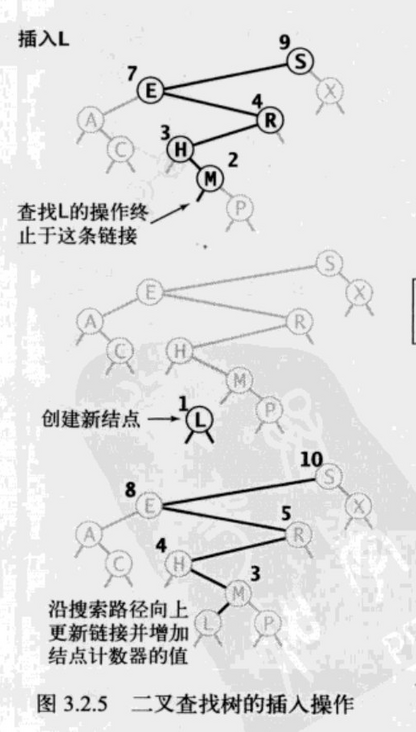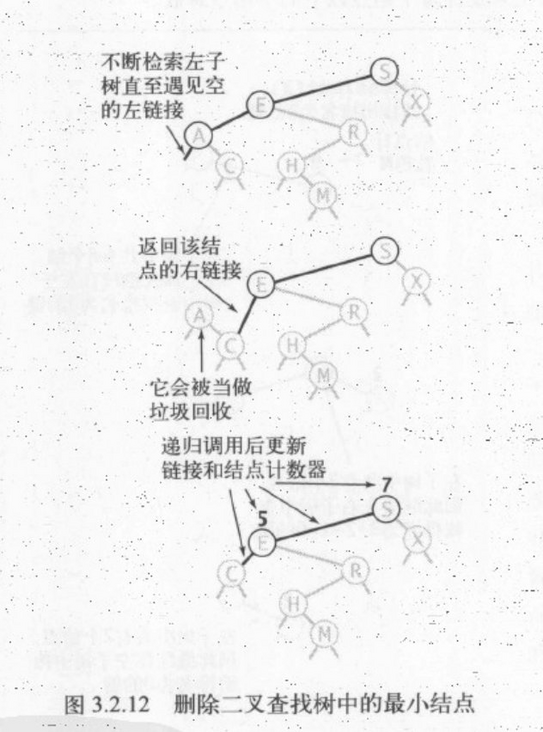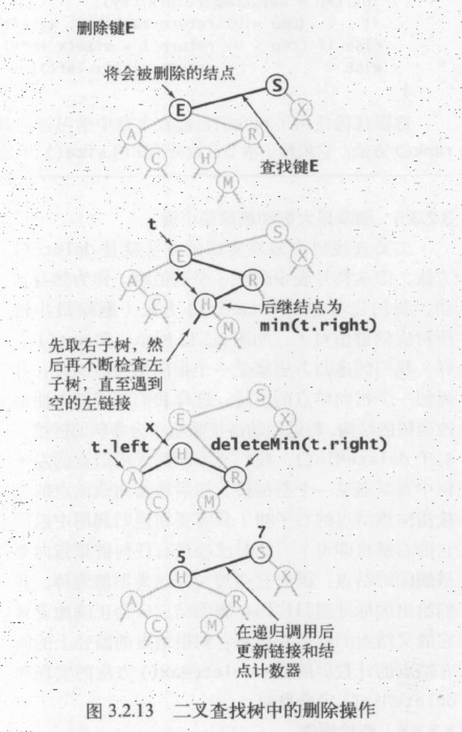# 基于二叉树实现Map

footballboy

0收藏

``````class Node {
private K key;
private V value;
private Node left;
private Node right;
private int size = 1;

public Node(K key, V value) {
this.key = key;
this.value = value;
}
}``````

size 记录当前节点所在子树的节点个数，计算方式：size=左子树的个数 + 1 + 右子树的个数

``````public interface Map<K, V> {
void put(K key, V value);

V get(K key);

void delete(K key);

int size();

Iterable<K> keys();

Iterable<TreeNode> nodes();

default boolean contains(K key) {
return get(key) != null;
}

default boolean isEmpty() {
return size() == 0;
}
}

public interface SortedMap<K extends Comparable<K>, V> extends Map<K, V> {
int rank(K key);

void deleteMin();

void deleteMax();

K min();

K max();
}``````

``````@Override
public V get(K key) {
if (Objects.isNull(key)) {
throw new IllegalArgumentException("key can not null");
}

Node node = get(root, key);
return Objects.isNull(node) ? null : node.value;
}

private Node get(Node node, K key) {
if (Objects.isNull(node)) {
return null;
}
int compare = key.compareTo(node.key);
if (compare > 0) {
return get(node.right, key);
} else if (compare < 0) {
return get(node.left, key);
} else {
return node;
}
}``````

``````@Override
public K max() {
Node max = max(root);
return max.key;
}

protected Node min(Node node) {
if (Objects.isNull(node.left)) {
return node;
}
return min(node.left);
}

protected Node max(Node node) {
if (Objects.isNull(node.right)) {
return node;
}
return max(node.right);
}````````````private Node put(Node node, K key, V value) {
if (Objects.isNull(node)) {
return new Node(key, value);
}

int compare = key.compareTo(node.key);
if (compare > 0) {
node.right = put(node.right, key, value);
} else if (compare < 0) {
node.left = put(node.left, key, value);
} else {
node.value = value;
}

node.size = size(node.left) + 1 + size(node.right);
return node;
}

private int size(Node node) {
if (Objects.isNull(node)) {
return 0;
}
return node.size;
}````````````@Override
public void deleteMin() {
root = deleteMin(root);
}

public Node deleteMin(Node node) {
if (Objects.isNull(node.left)) {
return node.right;
}
node.left = deleteMin(node.left);
node.size = size(node.left) + 1 + size(node.right);
return node;
}

@Override
public void deleteMax() {
root = deleteMax(root);
}

public Node deleteMax(Node node) {
if (Objects.isNull(node.right)) {
return node.left;
}
node.right = deleteMax(node.right);
node.size = size(node.left) + 1 + size(node.right);
return node;
}``````

1、从该节点的左子树中取出最大值或者是从右子树中取出最小值
2、用最大值或者最小值替换当前的节点
3、调用删除最大值或者删除最小值代码实现

``````@Override
public void delete(K key) {
root = delete(root, key);
}

private Node delete(Node node, K key) {
if (Objects.isNull(node)) {
return null;
}
int compare = key.compareTo(node.key);
if (compare > 0) {
node.right = delete(node.right, key);
} else if (compare < 0) {
node.left = delete(node.left, key);
} else {
if (Objects.isNull(node.left)) {
return node.right;
}
if (Objects.isNull(node.right)) {
return node.left;
}

Node max = max(node.left);
node.key = max.key;
node.value = max.value;

node.left = deleteMax(node.left);
}
node.size = size(node.left) + 1 + size(node.right);
return node;
}``````帖子
视频
声望
粉丝
最近发布
相关问题
社区精华内容